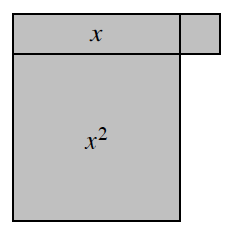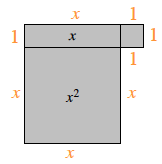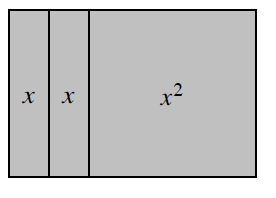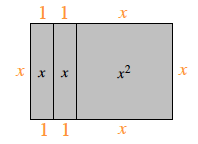Home > CC2MN > Chapter cc24 > Lesson cc24.3.2 > Problem4-93

4-93.

Copy the diagrams of algebra tiles below on your paper. Then find the perimeter of each shape. Homework Help ✎

1.The perimeter is the sum of all the sides. What is the value of each side?

Label each side piece.Now, add up all of the sides to find the perimeter.

$x+1+x+1+1+1+x+x$

Simplify to get $4x+4$.

1.Refer to part (a).

Label each side.